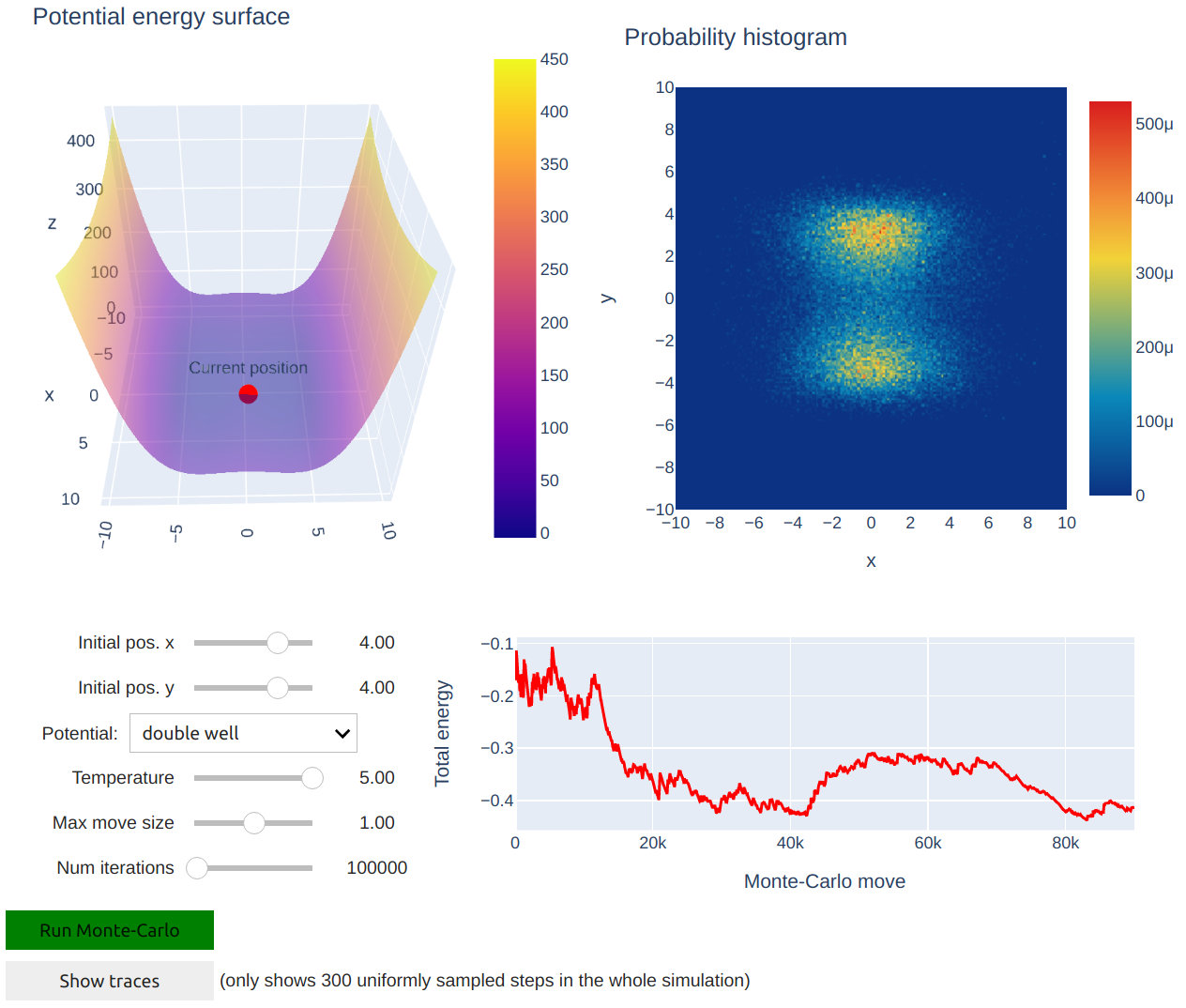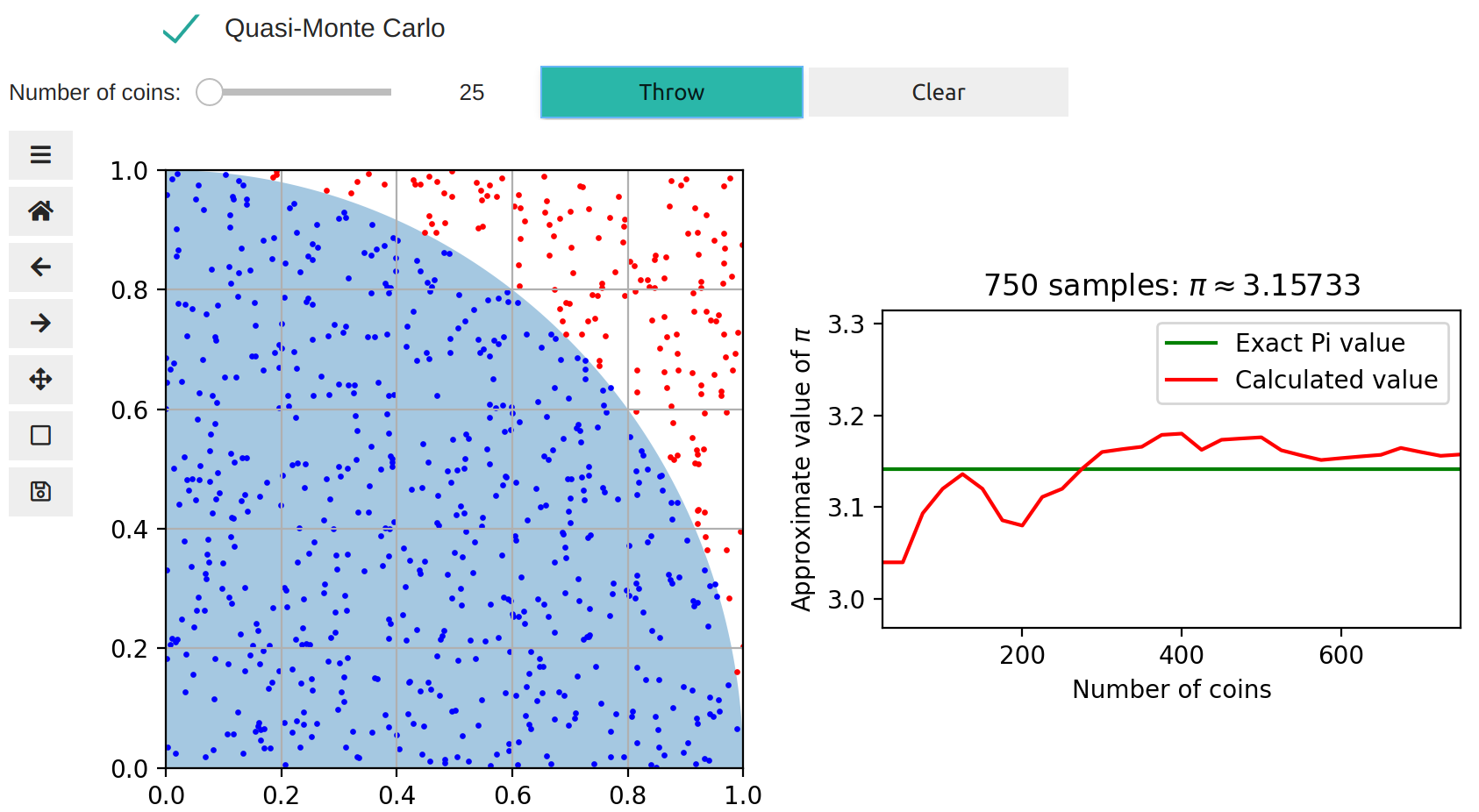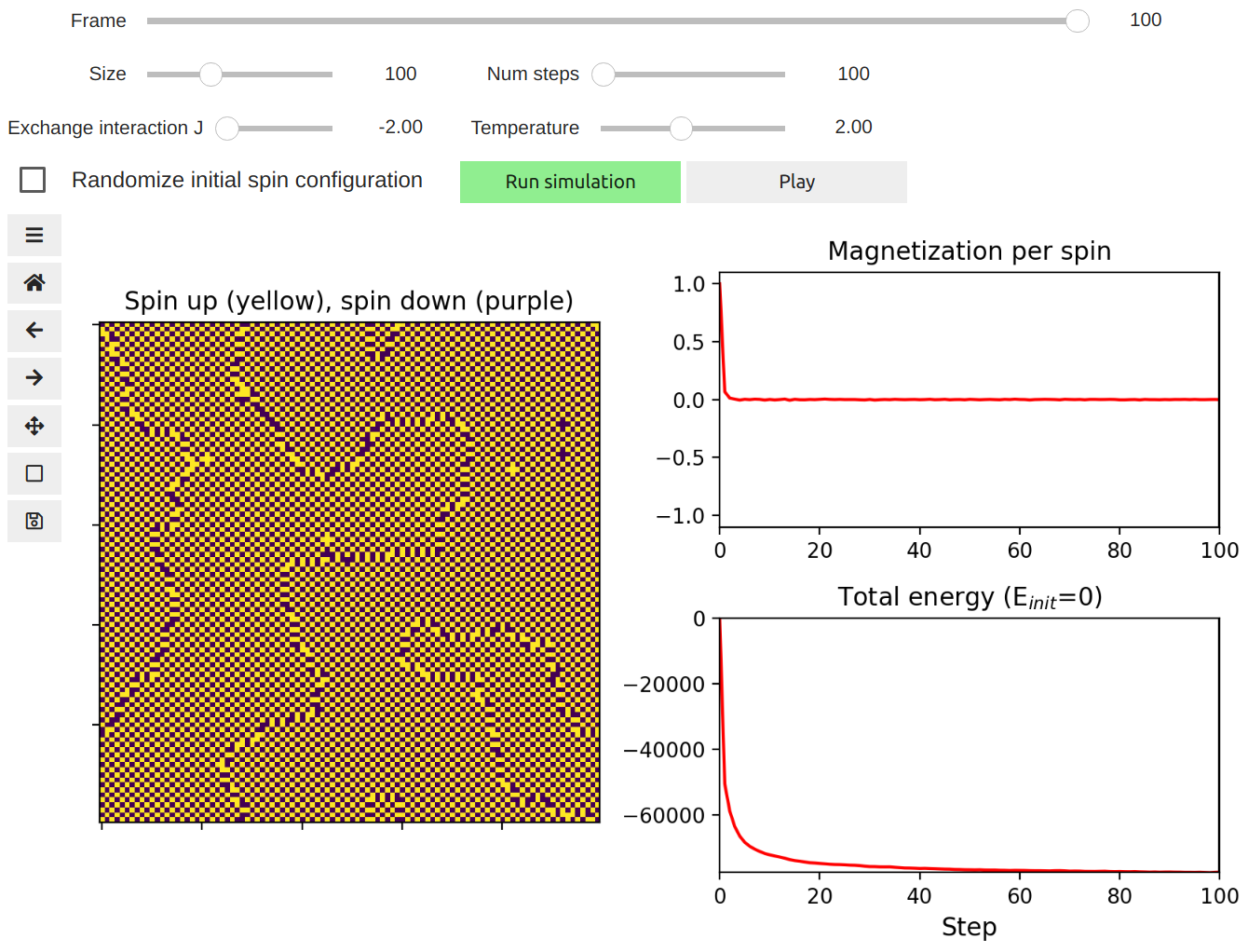# Statistical Mechanics¶

## 1. Monte Carlo Simulations to Sample the Canonical Distribution¶

Monte Carlo simulations are often used to sample the canonical distribution of a system given a potential energy surface. In this notebook, we demonstrate the use of the Metropolis-Hastings algorithm to find the global minimum of a two-dimensional potential energy surface.## 2. Monte Carlo Calculation of Pi¶

Monte Carlo methods encompass a large number of computational algorithms and methods, which rely on random number sampling to solve problems numerically. In this notebook, we introduce the Monte Carlo method by using a simple simulation to calculate the value of Pi.## 3. Ising Model in 2D¶

Magnetism is an important physical property of materials. The Ising model is a simple and successful model used to predict the net magnetization of a regular lattice of spins. Here, we have used Ising model to predict the ferromagnetic or antiferromagnetic behaviour of a square (2D) spin lattice.